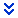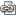# MAC 2105 Keiser University Week 2 Maths Worksheet

• February 05, 2022/

### Description

Need assistance in completed this week 2 of assignments.

• ### Week 2 Algebra TalkVariation is a great example of how mathematics is used to model and solve real-world problems. Many fields of study contain examples of the three different kinds of variation:

• Direct variation (for example, y = kx)
• Inverse variation (for example, y = k/x)
• Joint variation (for example, z = kxy)

Definition of Joint VariationJoint variation is the same as direct variation with two or more quantities.That is:Joint variation is a variation where a quantity varies directly as the product of two or more other quantities.Let’s first understand direct variation.Direct variation occurs when two quantities change in the same manner.That is:Increase in one quantity causes an increase in the other quantity.Decrease in one quantity causes a decrease in the other quantity.For example:The cost of a pencil and the number of pencils you buy.Buy more pay more….Buy less pay less.Direct variation between variables x and y can be expressed as:y = kx, where ‘ k‘ is the constant of variation and k ≠ 0.y = kxz represents joint variation. Here, y varies jointly as x and z.

### More Examples on Joint Variation

• y = 7xz, here y varies jointly as x and z.
• y = 7x2z3, here y varies jointly as x2 and z3.”

Math DictionaryThe variable is in the bottom of a fraction or in the denominator. (The Opposite of Direct Variation) In an inverse variation, the values of the two variables change in an opposite manner – as one value increases, the other decreases. For example, a biker traveling at 8 mph can cover 8 miles in 1 hour. If the biker’s speed decreases to 4 mph, it will take the biker 2 hours (an increase of one hour), to cover the same distance. Please start by addressing one or more of the following topics to get the discussion going:

1. How would you describe the difference between direct variation and joint variation in words? Use examples to help illustrate.
2. Pick a field of study of interest to you and give an example of variation in that field. Some possible areas to consider are: Economics, Finance, Geometry and Physics. Example: If your hourly pay rate is \$15/hour, then your weekly pay p is given by the formula p=15h where h is the number of hours worked in a week. So, your weekly pay varies directly as the number of hours worked in a week with the constant of variation = 15.
3. Most formulas are actually examples of variation. Pick a well-known formula and describe the type of variation as well as the constant of variation, k. Example: The area A of a triangle with base b and height h is given by the formula A=½bh. So, the area of a triangle varies jointly as the base and height (both direct) with the constant of variation = ½.
4. Give an example from real life where composite functions are utilized. For ideas, you may look it up on the internet or find examples in the book.

• ###Week 2 Weekly SummaryWhen posting here in the Weekly Summary section please do not post “answers only” but rather show how you obtained your answers in a step by step manner. If your solution is correct, it is in your best interest for us to see how you obtained the answer. If your solution is incorrect, showing your steps will help us to follow your process and we can then work together to find your mistake. No bonus points will be awarded for answers without explanations.A maximum of 5 or .25% bonus points will be awarded for 5 correct answers with step by step explanations.The weekly summary answers and explanations will be posted in the Announcement section by Friday of each week. Please try to work each problem without pasting and copying or you will defeat your purpose of learning. Credit will only be given for “original” work. Please feel free to study the explanations, learn the problem, and create your own step-by-step explanation for bonus points . You may post your explanations throughout the term (please remind your instructor in the General Questions thread to grade your problems if you have posted after Sunday).NOTE: You may submit a photo of your neat, hand-written work rather than a Word Document.

1. Find the vertex of the parabola f(x) = x2 – 16x + 63.
2. Find the x- and y-intercepts of the cubic function f(x) = (x+4)(2x-1)(x-1).
3. If f(x) = x2-2x-24 and g(x) = x2-x-30, find (f-g)(x).
4. If f(x) = x+4 and g(x) = 2x2-x-1, evaluate the composition (g o f)(2).
5. If f(x) = x+4 and g(x) = 2x2-x-1, find the composition (f o g)(x).
6. Write the following sentence as an equation: y varies directly as x.
7. Write the following sentence as an equation: x is directly proportional to y and is inversely proportional to the cube of z.
8. What are the zeros of the parabola: f(x) = x2 – 7x + 10 (that is, what are the x-intercepts or the points where the graph crosses the x-axis)?
9. What is the vertical asymptote of the rational function f(x) = 3x / (2x – 1)?
10. What is the horizontal asymptote of the rational function f(x) = 3x / (2x – 1)?